## Example Questions

### Example Question #1 : Matrix Combinations

Matthew, Emily, Cynthia, John, and Stephanie are all running for student council.  If three equivalent positions are available in this year's election, how many different leadership boards are possible?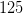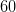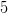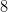Explanation:

One possible approach is to enumerate all possibilities using initials for names (ie. MEC, MEJ, MES, etc). If we recognize that order does not matter in this problem, we can also enter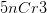into our calculators, and that will give us the answer of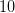.

### Example Question #1 : How To Find Combinations With A Matrix

Simplify.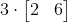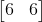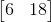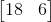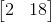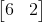Explanation:

When we are asked to simplify a matrix that is being multiplied by a constant we simply multiply each component of the matrix with the scalar factor that is on the outside of the matrix.

Therefore,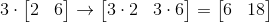### Example Question #31 : Matrices

At a sale, a necklace that was originally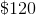is marked ten percent off. Cara has a coupon that will allow her to get ten percent off the discounted price. How much will Cara pay for the necklace if she uses the coupon?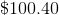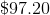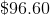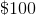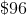Explanation:

Taking ten percent off ofgives us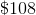,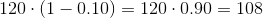.

Taking ten percent off of this amount gives us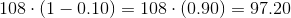.

NOTE: This problem cannot be solved by taking twenty percent off of.

### All ACT Math Resources